## Statistical Optimal Transport

Collaborators:

### Introduction

Optimal transport (OT) distances between probability measures or histograms, including the Earth Mover's Distance and Monge-Kantorovich or Wasserstein distance , have an increasing number of applications in statistics, such as unsupervised learning, semi-supervised learning, clustering, text classification, as well as in image retrieval, clustering, segmentation, and classification, and other fields, e.g. economics and finance or condensed matter physics.

Given a basis space and a transportation cost function, the OT approach defines a distance between two objects as a distance between two probability measures on a basis space. Consider an example of two grayscale images. In this case, the basis space can be defined as the pixel grid of this image. Then, for each pixel, the intensity can be seen as a mass situated at this pixel. Normalizing the intensity of each pixel dividing it by the total mass of the image, one obtains a discrete probability measure (or histogram) on the space of pixel grid. Then, the squared Euclidean distance between two pixels can be taken as a cost of transportation of a unit mass between these two pixels. Given two images, which are now modeled as probability measures, the goal is to transport the first image to the second. To do so, a transportation plan  is defined as a measure on the direct product of the basis pixel space by itself, i.e. a matrix with elements summing up to 1. Each row of this matrix corresponds to a pixel of the first image and tells what portion of mass is transported from this pixel to each pixel of the second image, see figure. At the same time, each column of this matrix corresponds to a pixel of the second image and tells what portion of mass is transported to this pixel from each pixel of the first image. Note that this definition is symmetric as the same transportation plan can be used to transport the second image to the first one.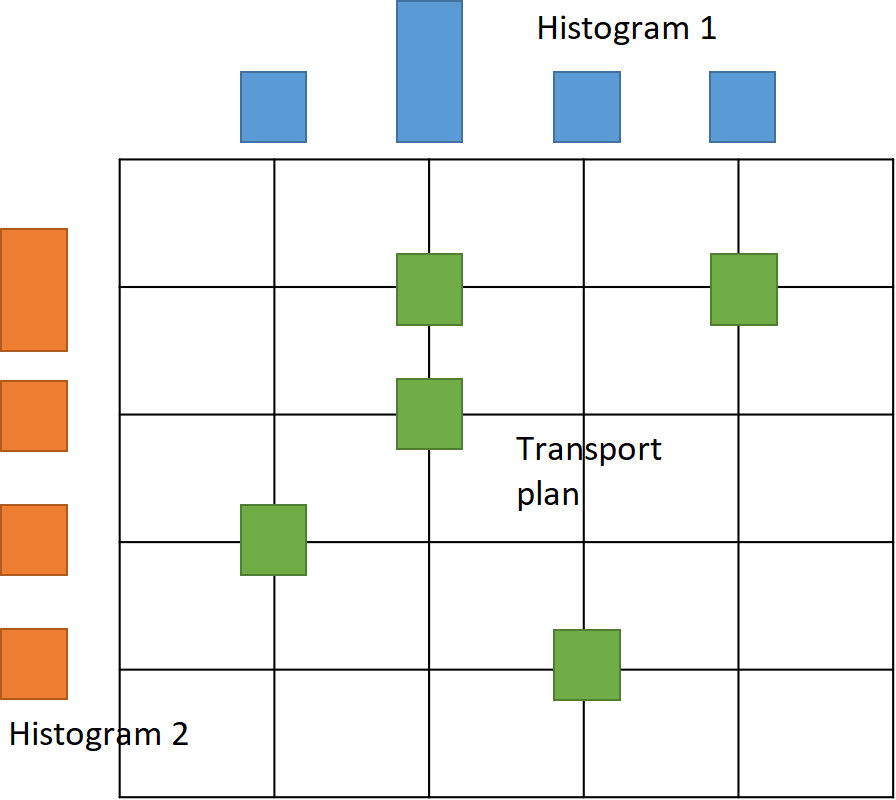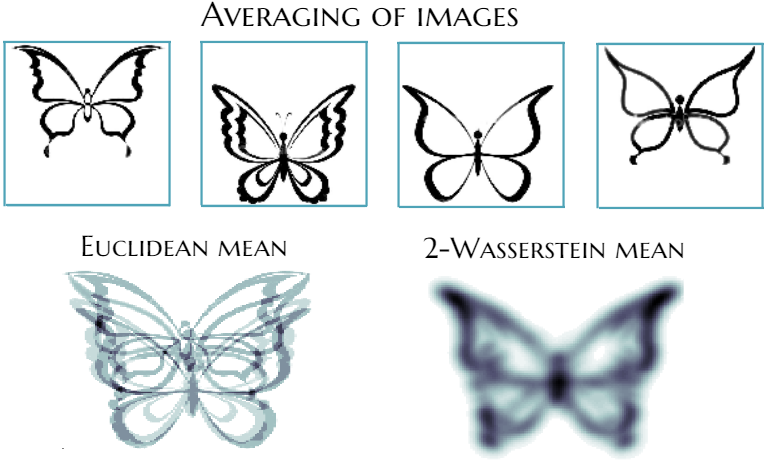Histograms and transportation plan (left). Euclidean and 2-Wasserstein barycenter of images (right).

Given squared euclidean distance as transportation cost (i.e., for each pair of pixels, the cost of transporting a unit of mass from a pixel in the first image to a pixel in the second) and transportation plan (i.e., for each pair of pixels, the mass which is transported from a pixel in the first image to a pixel in the second), one can calculate the total cost of transportation of the first image to the second by summing individual costs for each pair of pixels and finding the total cost. Now the the transportation plan can be varied in order to find the minimum possible total cost of transportation. The square root of this minimum transportation cost is called Wasserstein distance on the space of probability measures. This is a particular case of optimal transport distance. Optimal transport theory proves that this minimum is attained and is indeed a distance which satisfies all the distance axioms.

Besides images, these probability measures or histograms can model other real-world objects like videos, texts, genetic sequences, protein backbones, etc. The success of OT approach in applications comes for the price of intense computations, as the computation of the Wasserstein distance between two histograms requires to solve a large-scale optimization problem, which complexity scales quadratically with the size of histograms. Despite this computational complexity OT distance captures complicated geometry of these objects (images, videos, texts...) well, that makes it suitable for finding the mean in different tasks, unlike simple Euclidean distance, see the figure. As in the Euclidean space, when the mean object is defined as an object, minimizing the sum of Euclidean distances to all the objects in the set, the mean probability measure of a set of measures can be defined as a minimizer of the sum of squared Wasserstein distances to all the measures in the set. This mean object is called Wasserstein barycenter.

### Statistical Optimal Transport

Quite often modern data-sets appears to be too complex for being properly described by linear models. The absence of linearity makes impossible the direct application of standard inference techniques and requires a development of a new tool-box taking into account properties of the underlying space. We present an approach based on optimal transportation theory that is a convenient instrument for the analysis of complex data sets. The theory originates from seminal works of a french mathematician Gaspard Monge published at the end of 18th century. The work  investigates applicability of the classical resampling technique referred to as multiplier bootstrap for the case of 2-Wasserstein space. This line of research is continued by  where we investigate the convergence and concentration properties of the empirical barycenters and extend the setting to the Bures-Wasserstein distance. The obtained theoretical results are used for the introduction of Gaussian processes indexed by multidimensional distributions: positive definite kernels between multivariate distributions are constructed via Hilbert space embedding relying on optimal transport maps.

### Computational Optimal Transport

Calculating the Wasserstein barycenter of m measures is a computationally hard optimization problem which includes repeated computation of m Wasserstein distances. Moreover, in the large-scale setup, storage and processing of transportation plans, required to calculate Wasserstein distances, can be intractable for computation on a single computer. On the other hand, recent studies on distributed learning and optimization algorithms demonstrated their efficiency for statistical and optimization problems over arbitrary networks of computers or other devices with inherently distributed data, i.e., the data is produced by a distributed network of sensors or the transmission of information is limited by communication or privacy constraints, i.e., only limited amount of information can be shared across the network.

Motivated by the limited communication issue and the computational complexity of the Wasserstein barycenter problem for large amounts of data stored in a network of computers, we use state-of-the-art of computational optimal transport and convex optimization algorithms to propose a decentralized distributed algorithm to calculate an approximation to the Wasserstein barycenter of a set of probability measures. We solve the problem in a distributed manner on a connected and undirected network of agents oblivious to the network topology. Each agent locally holds a possibly continuous probability distribution, can sample from it, and seeks to cooperatively compute the barycenter of all probability measures exchanging the information with its neighbors. We adapt stochastic-gradient-type method and on each iteration each node of the network gets a stochastic approximation for the gradient of its local part of the objective, exchanges these stochastic gradients with the neighbours and makes a step. We show that as the time goes, the whole system comes to a consensus and each node holds a good approximation for the barycenter. The details can be found in . The output of the algorithm is illustrated in the following Figure.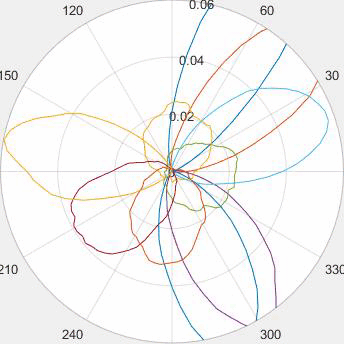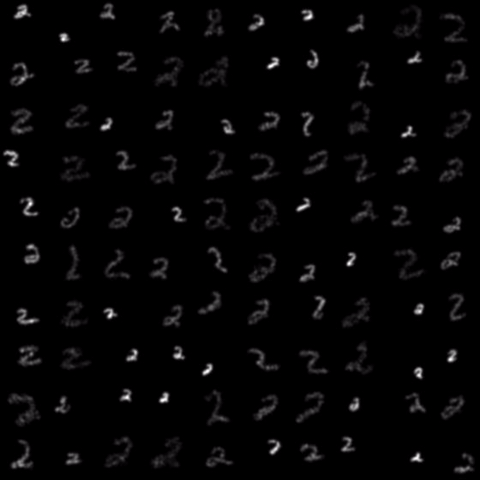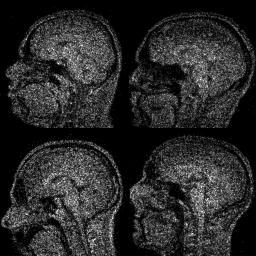Evolution of local approximation of the 2-Wasserstein barycenter on each node in the network for von Mises distributions (left), images from MNIST dataset (middle), brain images from IXI dataset (right). As the iteration counter increase, local approximations converge to the same distribution which is an approximation for the barycenter. Simulations made by César A. Uribe for the paper .

We also study complexity bounds of solving different types of optimal transport problems, e.g. approximating optimal transport distances  or approximating Wasserstein barycenter . Another topic is acceleration of alternating minimization algorithms and their application for optimal transport problems .

In the project "Optimal transport for imaging" within The Berlin Mathematics Research Center MATH+, following  we study also image segmentation using optimal transport distances as a fidelity term. A collaborator in this project is Prof. Dr. Michael Hintermüller, head of Research group 8 and Roman Kravchenko from HU Berlin.

### References

1. C. Villani. Optimal transport: old and new, volume 338. Springer Science & Business Media, 2008.
2. L. Kantorovich. On the translocation of masses. Doklady Acad. Sci. USSR (N.S.), 37:199-201, 1942.
3. J. Ebert, V. Spokoiny, and A. Suvorikova. Construction of non-asymptotic confidence sets in 2-Wasserstein space. arXiv:1703.03658, 2017.
4. A. Kroshnin, V. Spokoiny, and A. Suvorikova. Statistical inference for Bures-Wasserstein barycenters. arXiv:1901.00226, 2019.
5. P. Dvurechensky, D. Dvinskikh, A. Gasnikov, C. A. Uribe, and A. Nedic. Decentralize and randomize: Faster algorithm for Wasserstein barycenters. In S. Bengio, H. Wallach, H. Larochelle, K. Grauman, N. Cesa-Bianchi, and R. Garnett, editors, Advances in Neural Information Processing Systems 31, NIPS'18, pages 10783-10793. Curran Associates, Inc., 2018. arXiv:1802.04367.
6. P. Dvurechensky, A. Gasnikov, and A. Kroshnin. Computational optimal transport: Complexity by accelerated gradient descent is better than by Sinkhorn's algorithm. In J. Dy and A. Krause, editors, Proceedings of the 35th International Conference on Machine Learning, volume 80 of Proceedings of Machine Learning Research, pages 1367-1376, 2018. arXiv:1802.04367.
7. A. Kroshnin, N. Tupitsa, D. Dvinskikh, P. Dvurechensky, A. Gasnikov, and C. Uribe. On the complexity of approximating Wasserstein barycenters. In K. Chaudhuri and R. Salakhutdinov, editors, Proceedings of the 36th International Conference on Machine Learning, volume 97 of Proceedings of Machine Learning Research, pages 3530-3540, Long Beach, California, USA, 09-15 Jun 2019. PMLR. arXiv:1901.08686.
8. S. V. Guminov, Y. E. Nesterov, P. E. Dvurechensky, and A. V. Gasnikov. Accelerated primal-dual gradient descent with linesearch for convex, nonconvex, and nonsmooth optimization problems. Doklady Mathematics, 99(2):125-128, 2019.
9. N. Papadakis and J. Rabin. Convex histogram-based joint image segmentation with regularized optimal transport cost. Journal of Mathematical Imaging and Vision, 59(2):161-186, 2017.